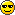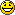# Subnet question

Hi guys,

I was going over a few practise questions i've found on the net and this was one of them..

You need to plan a custom subnet for the network for 6 Servers, 26 clients and future expansion for 5 more clients (so a total of 37) and the answers are...

a) 192.168.4.64/27
b) 192.168.4.32/27
c) 192.168.4.64/26
d) 192.168.4.32/26

Which answer would it be and why? I've always been a bit rusty with subnetting, once I have had this fully explained it will help me a great deal to pick it back up again as I find it quite hard to pick up from the MS book. Thanks

Another question, if you have a subnet of 255.255.255.255, working back one bit at a time you would get...

1 bits off 255.255.255.255 would be 255.255.255.254 = /31 = maximum number of clients?
2 bits off 255.255.255.255 would be 255.255.255.252 = /30 = " "
3 bits off 255.255.255.255 would be 255.255.255.248 = /29 etc
4 bits off 255.255.255.255 would be 255.255.255.240 = /28 etc
5 bits off 255.255.255.255 would be 255.255.255.224 = /27 etc
6 bits off 255.255.255.255 would be 255.255.255.192 = /26 etc
7 bits off 255.255.255.255 would be 255.255.255.128 = /25 etc
8 bits off 255.255.255.255 would be 255.255.255.0 = /24 etc

Is that right yes? If somebody could fill the maximum number of clients part of each line in for me that would help me get my head around this much easier. Cheers in advanceLuke

• Member Posts: 3,352 ■■■■□□□□□□
26 leaves 6 bits left. For clients, it's 2n-2. So 34 client bits are left.
27 leaves 5 bits left. for clients, it's 2n-2. So 23 client bits are left. This obviously doesn't satisfy the requirements for 6 servers, 26 clients, and scalability for 5 more users. So we know just narrowed down the answer to c or d.

So with a /26 we have a subnet mask of 255.255.255.192
11111111.11111111.11111111.11000000

Taking a look at the last bit, that bit value is in the placement of 64. This means our first subnet will start at 64. Since we're subnetting class C and borrowing 2 subnets from the last octet to work with, we have 2 bits to work with as subnet bits.

11
128 and 64

with subnets, it's 2n. Since we have 2 bits, that's 4 subnets to work with.
00
01
10
11

Our lowest subnet is 64 since it's in the 64 bit position.

“For success, attitude is equally as important as ability.” - Harry F. Banks
• Think i've just managed to work it out by finding the correct answer from a lancalc...

255, minus the last part of the subnet, then minus 1 for the broadcast 255 IP = maximum number of clients right?

1 bits off 255.255.255.255 would be 255.255.255.254 = /31 = maximum number of clients = 1
2 bits off 255.255.255.255 would be 255.255.255.252 = /30 = maximum number of clients = 2
3 bits off 255.255.255.255 would be 255.255.255.248 = /29 = maximum number of clients = 6
4 bits off 255.255.255.255 would be 255.255.255.240 = /28 = maximum number of clients = 14
5 bits off 255.255.255.255 would be 255.255.255.224 = /27 = maximum number of clients = 30
6 bits off 255.255.255.255 would be 255.255.255.192 = /26 = maximum number of clients = 62
7 bits off 255.255.255.255 would be 255.255.255.128 = /25 = maximum number of clients = 126
8 bits off 255.255.255.255 would be 255.255.255.0 = /24 = maximum number of clients = 254

Therefore, the answer to my original post would be c right?

9 bits off 255.255.255 would be 255.255.254.0 = /23 = maximum number of clients = 508
10 bits off 255.255.255 would be 255.255.248.0 = /22 = maximum number of clients = 1018
11 bits off 255.255.255 would be 255.255.240.0 = /21 = maximum number of clients = 2036

Am I right in thinking that you can just double the last number of clients available then add 2? Sorry for the boring rubbish above but i'm trying to get my head around it all.
• Member Posts: 3,352 ■■■■□□□□□□
Go read my last post.“For success, attitude is equally as important as ability.” - Harry F. Banks
• royal wrote:
26 leaves 6 bits left. For clients, it's 2n-2. So 34 client bits are left.
27 leaves 5 bits left. for clients, it's 2n-2. So 23 client bits are left. This obviously doesn't satisfy the requirements for 6 servers, 26 clients, and scalability for 5 more users. So we know just narrowed down the answer to a or b.

So with a /26 we have a subnet mask of 255.255.255.192
11111111.11111111.11111111.11000000

Taking a look at the last bit, that bit value is in the placement of 64. This means our first subnet will start at 64. Since we're subnetting class C and borrowing 2 subnets from the last octet to work with, we have 2 bits to work with as subnet bits.

11
128 and 64

with subnets, it's 2n. Since we have 2 bits, that's 4 subnets to work with.
00
01
10
11

Our lowest subnet is 64 since it's in the 64 bit position.

That's helped me out greatly, I figured out which it was out of the /26 and /27 but couldn't see why you would choose to start the IP range at 64 over 32. I now understand that you go by where the last bit is within the binary calculation i.e. 2nd along so 128-64 = .64Great stuff buddy, cheers.
Go read my last post.I'm such a slow typer, that by the time i'd put my second post up your reply was there. Well, it's either your too quick or i'm too slow :P